Question

Logic Circuits/Vlsi

21. Consider a ninth-order analog Butterworth lowpass filter H(s) with 3 dB cutoff frequency of 10 Hz.

(a) Determine and graph the pole locations ofH(s).

(b) Plot the magnitude and log-magnitude[0, 100] Hz range.responses over

(c) Determine frequencies at which the attenuation is 30 dB, 40 dB, and 50 dB.

\mathrm{VG} \simeq \sum_{k=0}^{K}|h[n]|^{2} . \quad K \gg 1

\mathrm{VG}=R_{0}^{2}+\sum_{k=1}^{N} \sum_{k=1}^{N} \frac{R_{k} R_{\ell}^{*}}{1-p_{k} p_{\ell}^{*}}Verified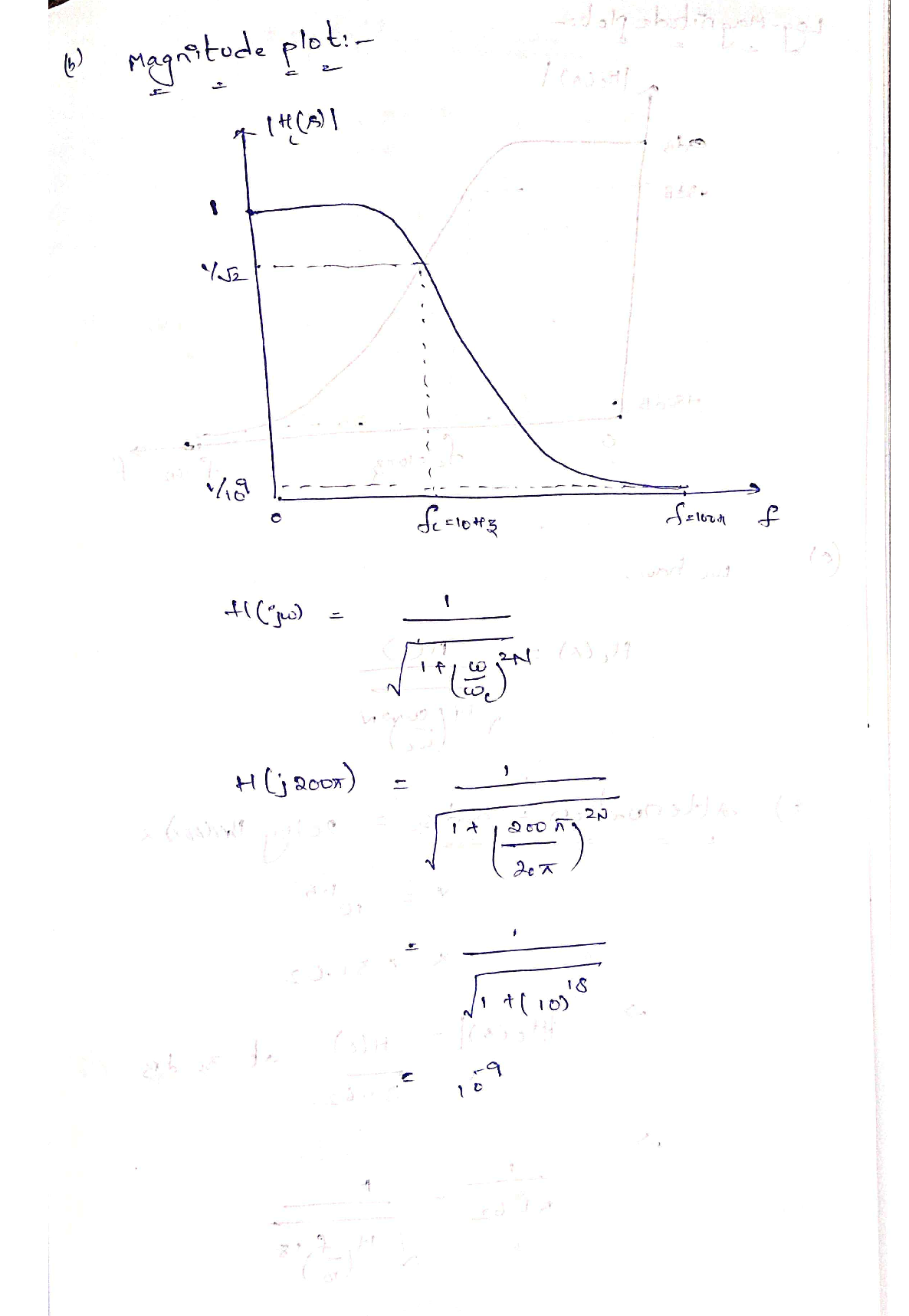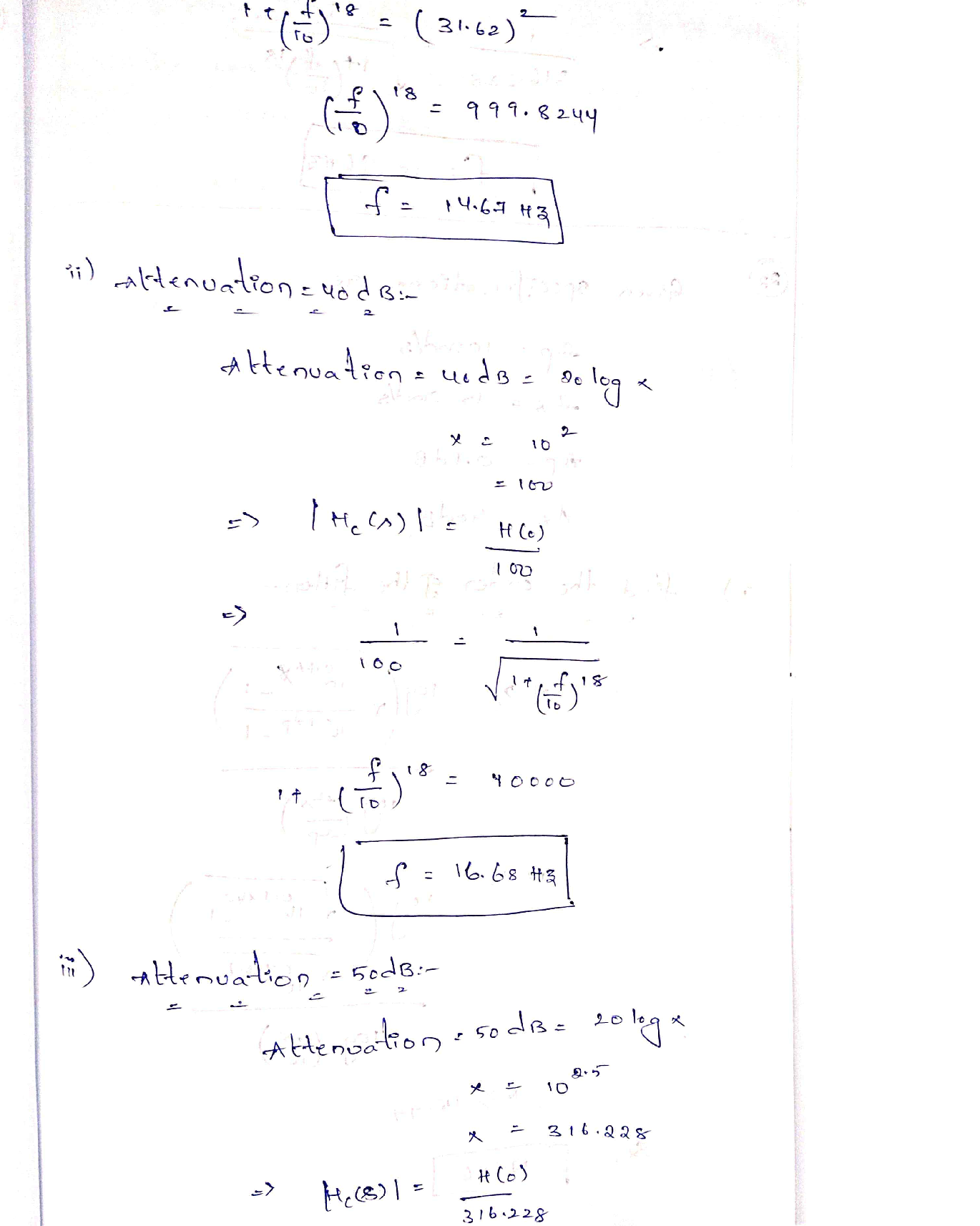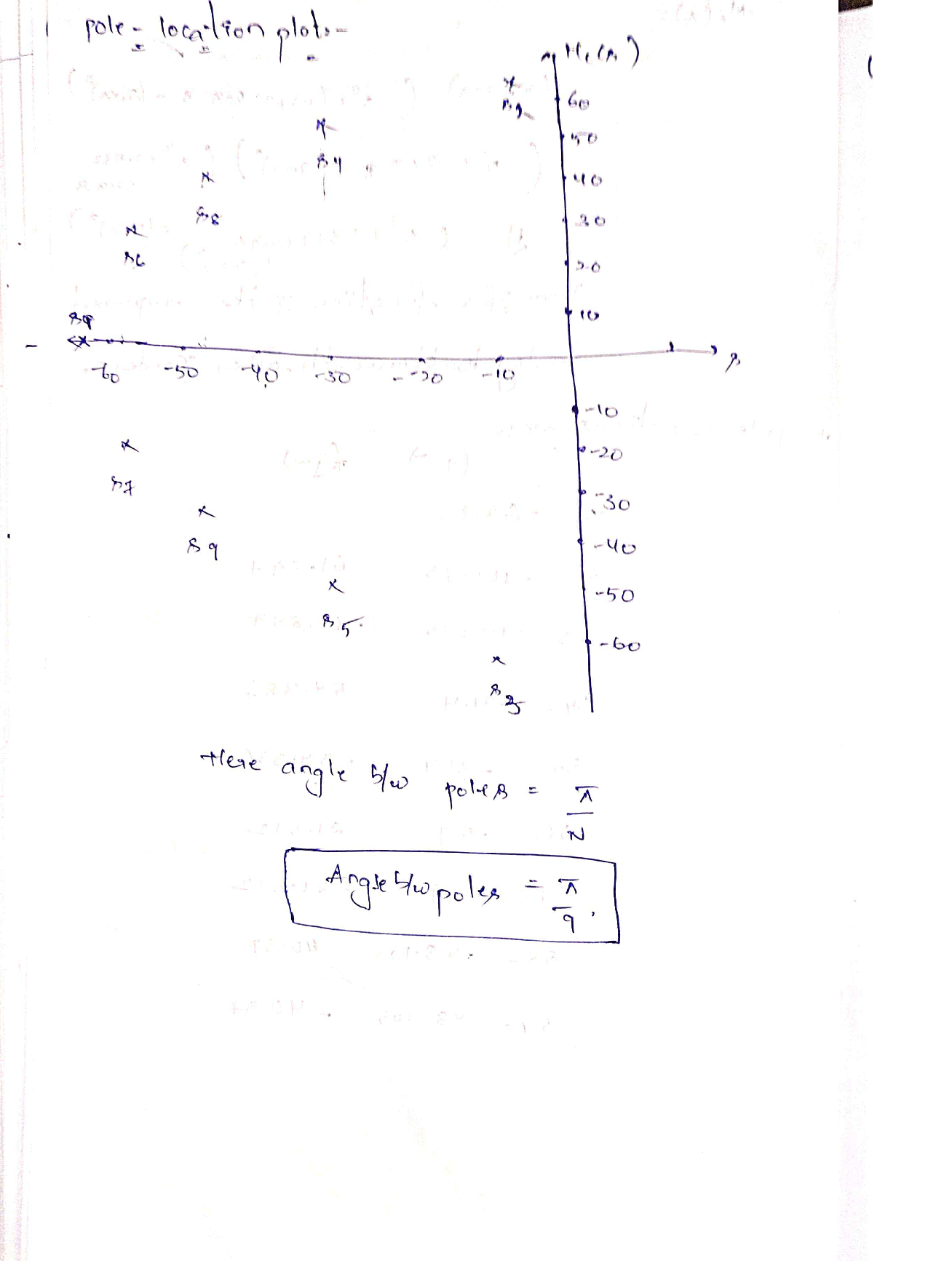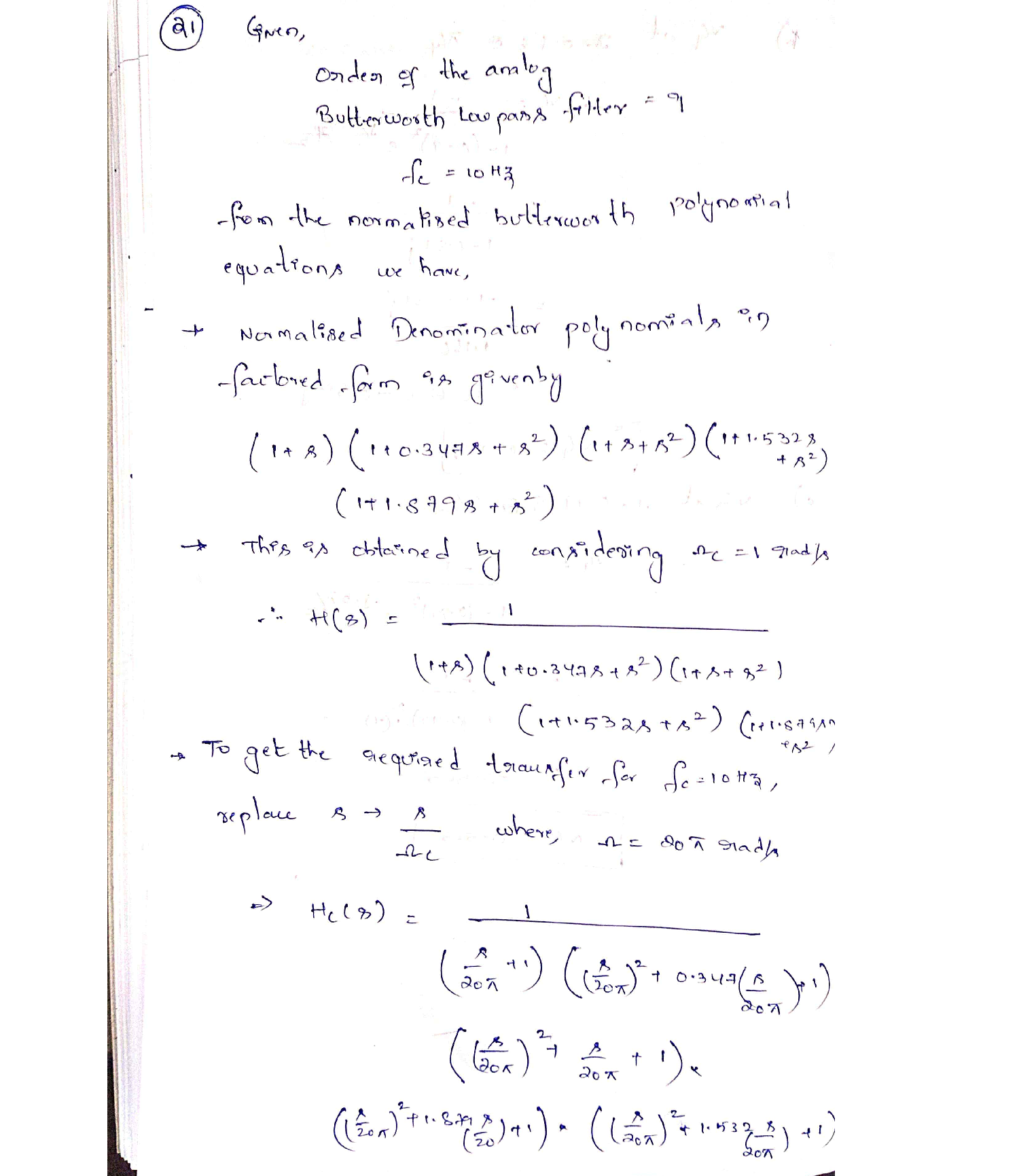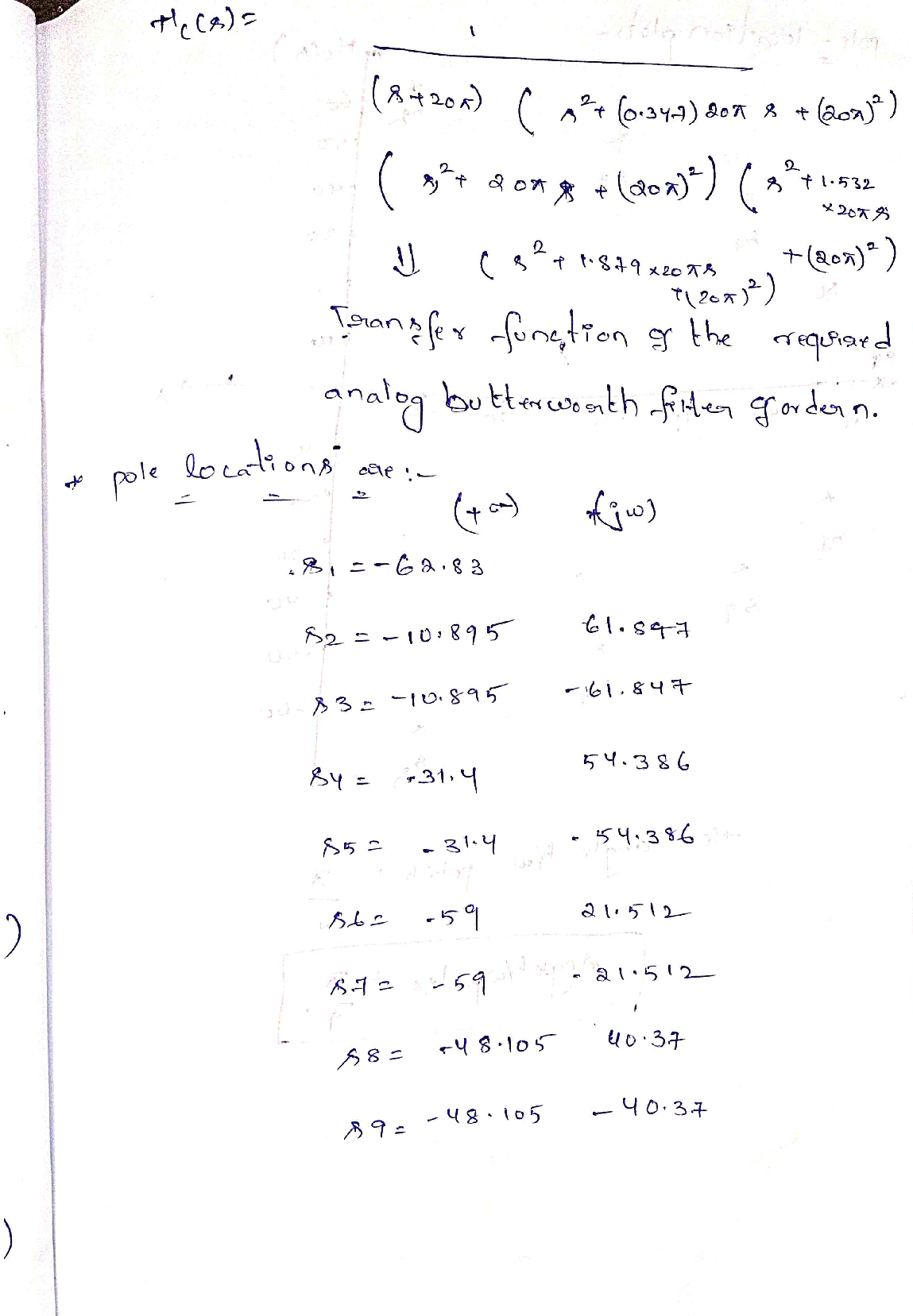### Question 44579Logic Circuits/Vlsi

Problem 7: (10 pts) Construct the truth table for the following circuit:

### Question 44578Logic Circuits/Vlsi

Problem 6: (5 pts) Design a gate circuit that outputs a 1 if the number of l's on the four inputs A, B, C, D is odd using 2-input XOR gates.

### Question 44577Logic Circuits/Vlsi

Problem 5: (10 pts) The security alarm system you designed in Problem 4 has an issue that it accidentally gets triggered by pets. You consider improving the system by triggering an alarm only if at least two motion sensors are activated at the same time. Show a truth table that has the four motion sensors as inputs and an alarm trigger as the output which shows how to implement the improved security system design.

### Question 44576Logic Circuits/Vlsi

Problem 4: (10 pts) You are asked to build a digital logic circuit for a security alarm system. The system has four Motion Sensors which indicate the presence of an intruder. Each individual motion sensor should be able to trigger an alarm. The system should be completely disabled via a Master Switch. In addition, the siren, the flashlight and the automated call to the security should have separate enable switches. The inputs and outputs are specified as follows:company

### Question 44575Logic Circuits/Vlsi

Problem 3: (3+3+4 pts) Show a truth table for each of the following circuits:

### Question 44574Logic Circuits/Vlsi

Problem 2: (10 pts) Determine, using a truth table, whether the two circuits are equivalent interms of logic.

### Question 44573Logic Circuits/Vlsi

Problem 1: (2 + 2 + 2 + 2 + 2 pts) Show a truth table for each of the following circuits:

### Question 44487Logic Circuits/Vlsi

7. Draw the circuit and truth tables for the following equations
(a) (a * č) + (b* c)
(b) (a* b* č) + (ā * b* €)

### Question 44486Logic Circuits/Vlsi

Convert the following hexadecimal numbers into binary and decimal
(a) 0×45FD
(b) ОхАВ24
(c) 0x1256

### Question 44485Logic Circuits/Vlsi

5. Convert the following binary numbers into heaxadecimal(o) 1111 01011100 0001
(a) 1111 0101 1100 0001
(b) 0011 0111 0011 1111
(c) 0110 1010 1101 0100

### Submit query